##### Math Word Problems For Dummies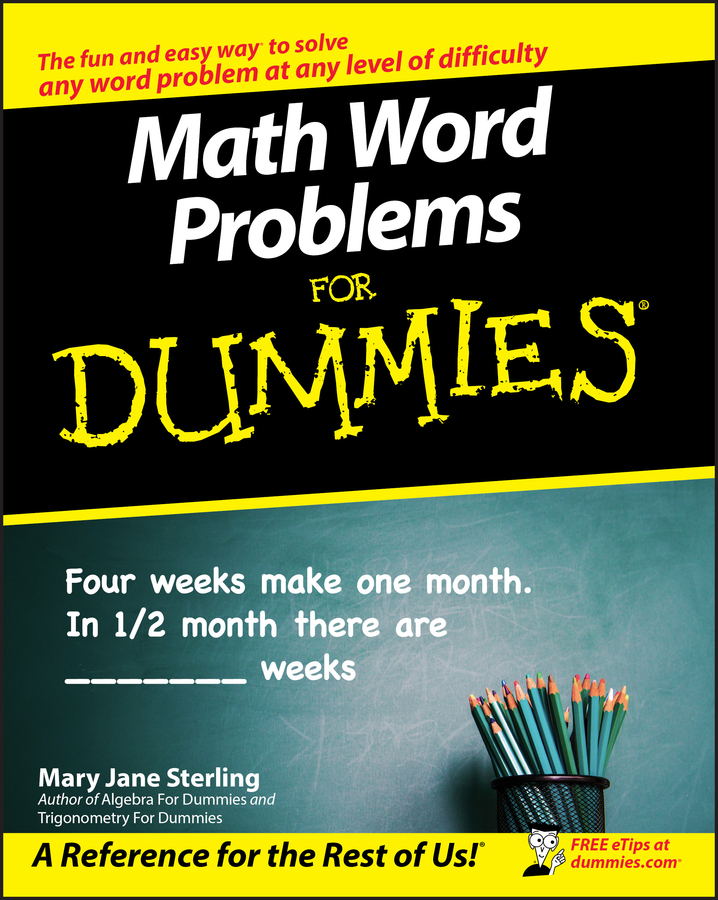Say you’re filling up your swimming pool and you know how fast water is coming out of your hose, and you want to calculate how fast the water level in the pool is rising. You know one rate (how fast the water is being poured in), and you want to determine another rate (how fast the water level is rising). These rates are called related rates because one depends on the other — the faster the water is poured in, the faster the water level will rise. In a typical related rates problem, the rate or rates you’re given are unchanging, but the rate you have to figure out is changing with time. You have to determine this rate at one particular point in time.

Here’s a garden-variety related rates problem. A trough is being filled up with swill. It’s 10 feet long, and its cross-section is an isosceles triangle that has a base of 2 feet and a height of 2 feet 6 inches (with the vertex at the bottom, of course). Swill’s being poured in at a rate of 5 cubic feet per minute. When the depth of the swill is 1 foot 3 inches, how fast is the swill level rising?

1. Draw a diagram, labeling the diagram with any unchanging measurements and assign variables to any changing things.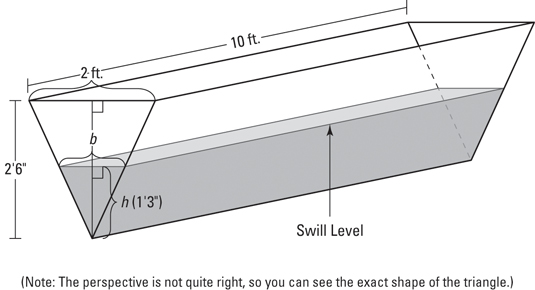It’s important that your figure show the unchanging dimensions of the trough — 2 feet, 2 feet 6 inches, and 10 feet — and that you do not give these dimensions variable names like l for length or h for height. And note that the changing things — the height (or depth) of the swill and the width of the surface of the swill (which gets wider while the swill gets deeper) — must have variable names, like h for height and b for base. (It’s called the base instead of the width because it’s the base of the upside-down triangle shape made by the swill.) The volume of the swill is also changing, so you can call that V, of course.

2. List all given rates and the rate you’re asked to figure out as derivatives with respect to time.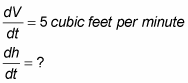3. Write down the formula that connects the variables in the problem: V, h, and b.

The formula for the volume of a right prism (the shape of the swill in the trough) is

V = (area of base)(height)

This “base” is the base of the prism (the whole triangle at the end of the trough), not the base of the triangle, which is labeled b in the figure. Also, this “height” is the height of the prism (the length of the trough), not the height labeled h in the figure.

The area of the triangular base equals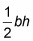and the “height” of the prism is 10 feet, so the formula becomes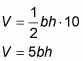4. Find an equation that relates the unwanted variable, b, to some other variable in the problem so you can make a substitution that leaves you with only V and h.

The triangular face of the swill in the trough is similar to the triangular face of the trough itself, so the base and height of these triangles are proportional. Thus,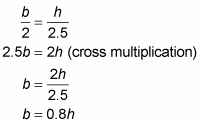Keep in mind that similar triangles come up a lot in related rates problems. Look for them whenever the problem involves a triangle, a triangular prism, or a cone shape.

Now substitute 0.8h for b in your formula from Step 3.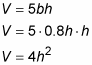5. Differentiate this equation with respect to t.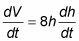6. Substitute known values for the rate and variable in the equation from Step 5 and then solve.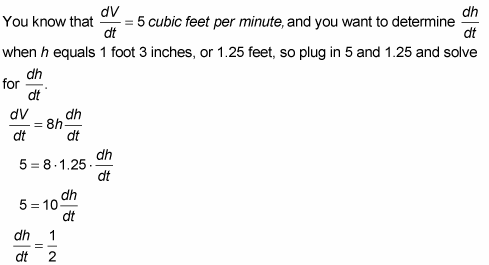That’s it. The swill’s level is rising at a rate of 1/2 foot per minute when the swill is 1 foot 3 inches deep. Dig in!